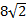You can only add radicals that have the same radicand (the same expression inside the square root). Here's how to add them: 1) Make sure the radicands are the same. If not, you cannot add them. 2) Add the outside numbers 3) Keep the radicand (the inside) the same. Let's examine a few examples. 1)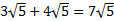Since the radicands match, all we have to do is add 3+4 and keep thethe same. 2)There is an understood one in front of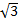, so 5+1=5 andstays the same. 3)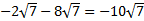Don't let negatives through you off. -2-8=-10 and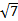stays the same. 4)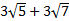This one cannot be added since the radicands do not match. This is the final answer. 5)At first glance, it appears this one cannot be added. However,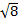can be simplified.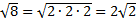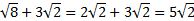Practice: Add the radicals. 1)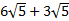2)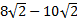3)4)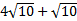5)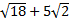Answers: 1)2)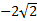3)4)5)